# epicycloid

## rouletteThe curve is formed by the locus of a point, attached to a circle, that rolls on 1) the outside of another circle 2). In the curve's equation the first part denotes the relative position between the two circles, the second part denotes the rotation of the rolling circle.

The value of the constant b determines the starting point in relation to the circle:

The isoptic of the ordinary epicycloid is an epitrochoid.

The curve has a closed form when the ratio of the rolling circle and the other circle (a) is equal to a rational number. When giving this ratio its simplest form, the numerator is the number of revolutions around the resting circle, before the curve closes. The denominator is the number of rotations of the rolling circle before this happens.
In this rational case the curve is algebraic, otherwise transcendental.

A epicycloid with parameters a and b is the same as a hypocycloid with parameters a+1, 1/b.

## (ordinary) epicycloid

The epicycloid curves have been studied by a lot of mathematicians around the 17th century: D³rer (1515), Desargues (1640), Huygens (1679), Leibniz, Newton (1686), de L'H¶pital (1690), Jakob Bernoulli (1690), la Hire (1694), Johann Bernoulli (1695), Danilel Bernoulli (1725) and Euler (1745, 1781).

Apollonius of Perga (about 200 BC), had the idea to describe the celestial movements as combinations of circular movements. It was Hipparchos of Nicaea (about 150 BC), the greatest astronomer in Greek antiquity, who worked out this theory in detail. The results did become famous by the books of Ptolemy (about 150 AD). The earth is thought as standing in (or nearby) a celestial center, around which the other celestial bodies rotate. The combination of the rotation of the earth and the planet's rotation around her makes an epicycloid. This geocentric theory should be the accepted theory for almost 2000 years. The heliocentric theory (as constructed by Copernicus), was also discussed by the Greek, but refused for emotional reasons.

Some relations with other curves:

• the radial and the pedal (with the center as pedal point) of the curve is the rhodonea
• the evolute of an epicycloid is a similar epicycloid, but smaller in size

The curve is a cycloidal curve.

There are some epicycloids that have been given an own name:

• a = 1/5: the ranunculoid
This curve has been named after the buttercup genus Ranunculus by Madachy (1979).
• a = 1/2: the nephroid
• a = 1: the cardioid

For 1 = 1/n, n gives the number of cusps.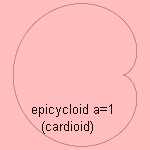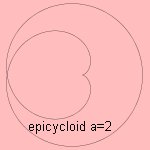## epitrochoid3)

Now the point being followed is not lying on the rolling circle. When the point lays outside the circle (b>1), the curve is called a prolate epicycloid. When the point lays inside the rolling circle (b<1), the curve is called a curtate epicycloid.
The variable b is the ratio of the distance from the starting point to the center of the rolling circle, and the radius of that circle.

With a spirograph set many epitrochoids can be drawn.

In D³rer's 'Instruction in measurement with compasses and straight edge' (1525) occurs an example of an epitrochoid. He called them spider lines because of the form of the construction lines he used.
Other mathematicians who studied the curves were: la Hire, Desargues and Newton.

And now some examples of epitrochoids: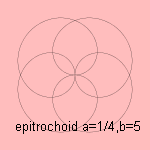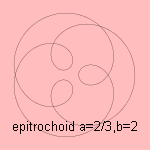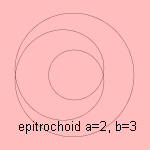When the two circles have equal radius (a=1), the epitrochoid is a limańon.

notes

1) Epi = on

2) Let a circle with radius r roll on the outside of a circle with radius R. Take as center of the coordinate system the center of the rolled circle. Now let the starting point be on a distance b r from the center of the rolling circle. Then the coordinates of the epicycloid as a function of the rolled angle t are: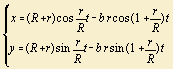3) Trochus (Lat.) = hoop.
Sometimes the meaning of epicycloid and epitrochoid is interchanged: epitrochoid for the general case, epicycloid only for the situation that the starting point is lying on the circle.